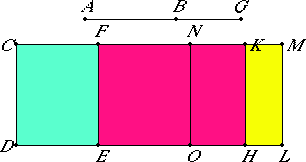# Proposition 99

The square on a second apotome of a medial straight line applied to a rational straight line produces as breadth a third apotome.

Let AB be a second apotome of a medial straight line, and CD rational, and to CD let there be applied CE equal to the square on AB producing CF as breadth.

I say that CF is a third apotome.

X.75

Let BG be the annex to AB, therefore AG and GB are medial straight lines commensurable in square only which contains a medial rectangle.Apply CH, equal to the square on AG, to CD producing CK as breadth, and apply KL, equal to the square on BG, to KH producing KM as breadth. Then the whole CL equals the sum of the squares on AG and GB. Therefore CL is also medial.

X.22

And it is applied to the rational straight line CD producing CM as breadth, therefore CM is rational and incommensurable in length with CD.

II.7

Now, since the whole CL equals the sum of the squares on AG and GB, and, in these, CE equals the square on AB, therefore the remainder LF equals twice the rectangle AG by GB.

Bisect FM at the point N, and draw NO parallel to CD. Then each of the rectangles FO and NL equals the rectangle AG by GB.

But the rectangle AG by GB is medial, therefore FL is also medial.

X.22

And it is applied to the rational straight line EF producing FM as breadth, therefore FM is also rational and incommensurable in length with CD.

Since AG and GB are commensurable in square only, therefore AG is incommensurable in length with GB. Therefore the square on AG is also incommensurable with the rectangle AG by GB.

X.13

But the sum of the squares on AG and GB is commensurable with the square on AG, and twice the rectangle AG by GB with the rectangle AG by GB, therefore the sum of the squares on AG and GB is incommensurable with twice the rectangle AG by GB.

But CL equals the sum of the squares on AG and GB, and FL equals twice the rectangle AG by GB, therefore CL is also incommensurable with FL.

VI.1

But CL is to FL as CM is to FM, therefore CM is incommensurable in length with FM.

X.73

And both are rational, therefore CM and MF are rational straight lines commensurable in square only, therefore CF is an apotome.

I say next that it is also a third apotome.

Since the square on AG is commensurable with the square on GB, therefore CH is also commensurable with KL, so that CK is also commensurable with KM.

Since the rectangle AG by GB is a mean proportional between the squares on AG and GB, CH equals the square on AG, KL equals the square on GB, and NL equals the rectangle AG by GB, therefore NL is also a mean proportional between CH and KL. Therefore CH is to NL as NL is to KL.

But CH is to NL as CK is to NM, and NL is to KL as NM is to KM, therefore CK is to MN as MN is to KM. Therefore the rectangle CK by KM equals the square on MN, that is, to the fourth part of the square on FM.

X.17

Since, then, CM and MF are two unequal straight lines, and a parallelogram equal to the fourth part of the square on FM and deficient by a square figure has been applied to CM, and divides it into commensurable parts, therefore the square on CM is greater than the square on MF by the square on a straight line commensurable with CM.

X.Def.III.3

And neither of the straight lines CM nor MF is commensurable in length with the rational straight line CD set out, therefore CF is a third apotome.

Therefore, the square on a second apotome of a medial straight line applied to a rational straight line produces as breadth a third apotome.

Q.E.D.

## Guide

This proposition is used in X.111.